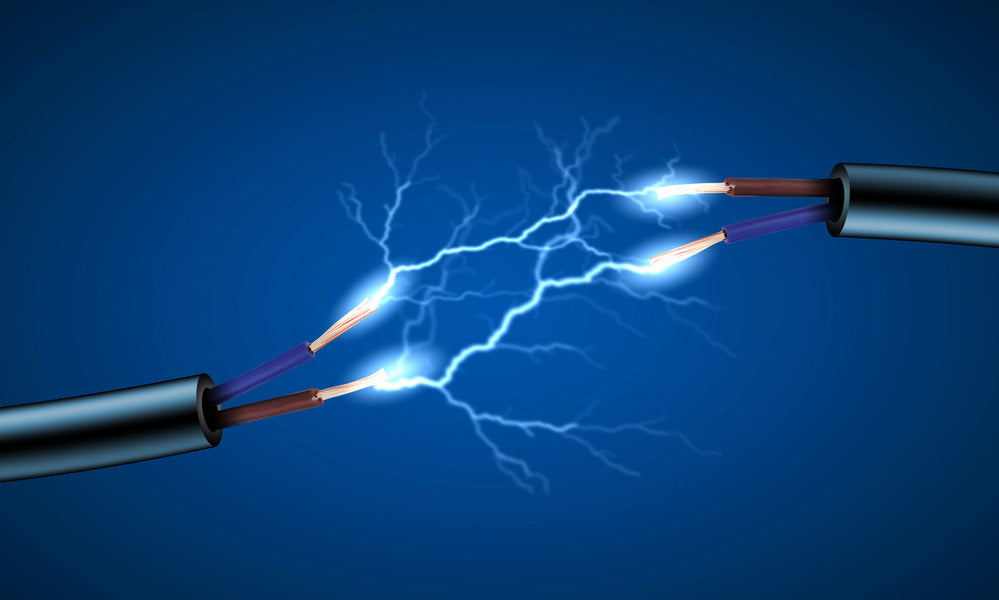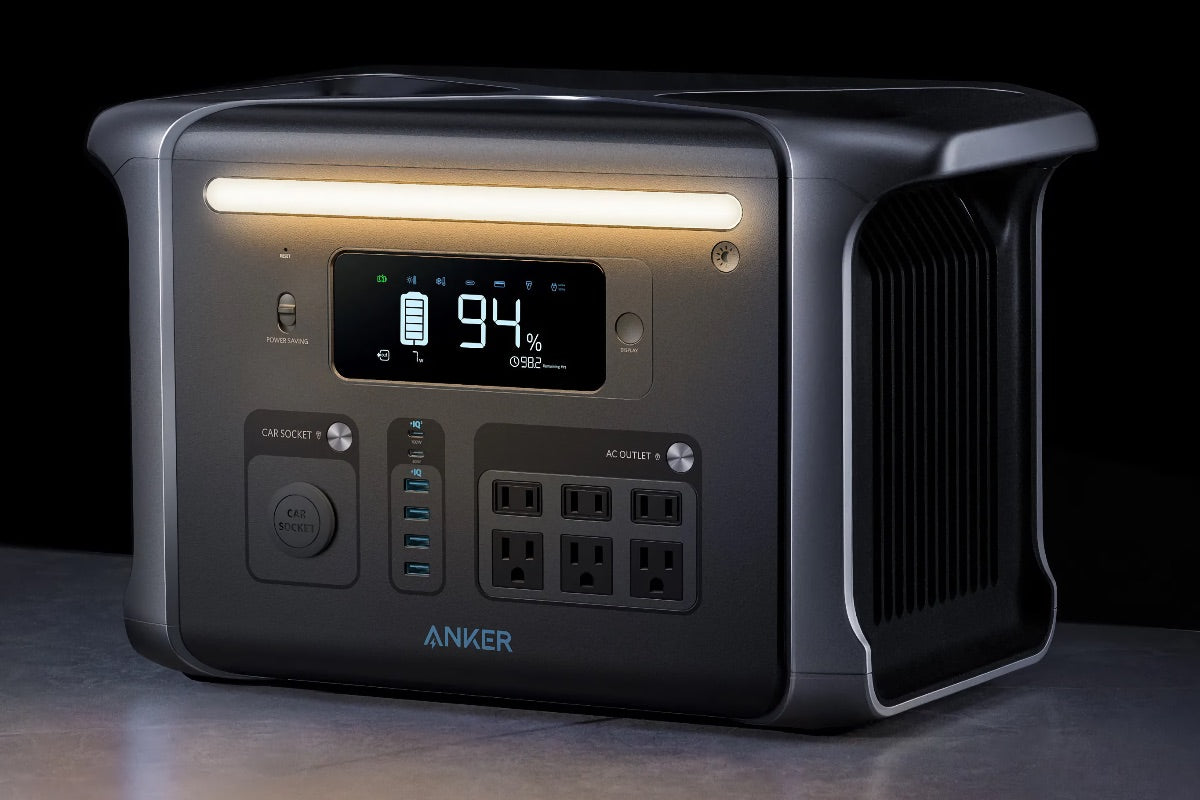///
Demystifying Electricity: Interplay of Volts, Watts to Amps

# Demystifying Electricity: Interplay of Volts, Watts to Amps

In the realm of electricity, three fundamental units of measurement are essential to understanding the flow and consumption of electrical energy: Amps, Watts, and Volts. These units play a crucial role in quantifying and describing various electrical phenomena. In this article, we will learn the distinction among these three units, what each measurement represents, and how they are used in the electrical world. Furthermore, we will debunk some common misconceptions about Amps, Watts, and Volts. Let's get started!## What is an Amp, Watt, and Volt in the Electrical World

In the electrical world, Watt (W), Ampere (Amp), and Volt (V) are fundamental units that represent different aspects of electrical energy. They are essential for understanding and working with electrical systems.

1. Watt (W)

A Watt is the unit of measurement for electrical power. It's the rate at which energy is used or generated. It is named after James Watt, a Scottish inventor and mechanical engineer. One Watt equates to one joule of energy per second. In electrical systems, power (Watts) is calculated by multiplying voltage (Volts) by current (Amps).

1. Ampere (Amp)

An Ampere, often shortened to Amp, is the unit of measurement for electrical current. It shows the flow of electrons through a conductor or circuit. One Ampere is equivalent to the flow of one coulomb of charge per second.

1. Volt (V)

A Volt is a unit of quantification for electrical potential difference, also known as voltage. It is named after Alessandro Volta, an Italian physicist and inventor. Voltage is a force that pushes electrons through a conductor or circuit, measured in Volts. One Volt equates to the potential difference when one joule (1 J) of energy is used to move one coulomb (1 C) of charge between two points.

## How Do Amps, Watts, and Volts Differ from Each Other

Amps, Watts, and Volts are all different units used to describe different aspects of electricity:

• Amps(Amperes) measure the flow of electrical current (charge) through a circuit.
• Watts measures the rate of energy consumption or generation, also known as power.
• Voltsmeasure the force or potential difference that drives the flow of electrons through a circuit.

These three units are related through the formula: Power (Watts) = Voltage (Volts) x Current (Amps)

## What Does Each Measurement Represent and How Are they Used

1. Watts (Power): The Product of Work and Electron Flow

Power is defined as the product of work done and the number of electrons traveling through a circuit in a given time. It is measured in watts (W) and can be calculated using the formula P = VI, where P represents power, V is voltage, and I is current. In electrical systems, power determines the efficiency and effectiveness of energy conversion and transmission. By understanding power, you can optimize the performance of electrical devices and systems.

1. Amps (Current): The Flow of Electricity

Current represents the flow of electricity through an electrical circuit. For example, if there's a current running through your hand, electricity will continue to flow until it's interrupted at another point in your body. Current is measured in amps (A), with one amp equal to 0.001 kilowatts per hour (kWh).

1. Volts (Voltage): The Electromotive Force

Voltage measures the pressure a power source requires to push charged electrons through a conducting loop, enabling the circuit to supply power to various devices. To calculate voltage, you can use Ohm's law, which states that voltage (V) is equal to the product of current (I) and resistance (R): V = IR.

## Common Misconceptions About Amps, Watts, and Volts

Here are the top 3 common misconceptions about Amps, Watts, and Volts:

1. You Can Interchange Voltage and Current Ratings for Devices

Each electrical device is designed to operate at a specific voltage and current range. Using a device outside of its intended voltage or current range can lead to reduced performance, damage, or even dangerous situations such as fires or electrical shock.

1. Higher Voltage Means More Power

While it is true that higher voltage can result in more power, it is important to remember that power is the product of both voltage and current. A higher voltage with a lower current can result in the same power output as a lower voltage with a higher current. The power depends on the combination of both voltage and current.

1. More Amps are Always Better

The amount of current (amps) needed depends on the specific application. In some cases, a higher current may be necessary to power a device or complete a task. However, in other cases, a higher current can be dangerous and lead to overheating or damage to electrical components. It is important just to use the appropriate amount of current for each application.

## Examples to better explained Amps, Watts, and Volts

Amps, Watts, and Volts are essential units of measurement in the world of electricity. To better understand these terms, let's use the Anker 757 PowerHouse as an example.

The Anker 757 PowerHouse is a long-lasting portable power station. With a 1229Wh capacity and 1500 wattage, it's perfect for powering essential appliances during trips. Moreover, it is equipped with multiple output options, including AC outlets, USB ports, and a 12V car socket.

Now, let's break down the concepts of Amps, Watts, and Volts using the Anker 757 PowerHouse as a reference.

• Amps

Amps measure the flow of electric current through a conductor. Think of it as the quantity of water flowing through a pipe. In the case of the Anker PowerHouse 757, it has a maximum output current of 10A for its 12V DC output, meaning it can provide up to 10 Amps of current to devices connected to that output.

• Volts

Volts measure the electric potential difference between two points in a circuit. It can be described as the pressure that pushes the electric current (Amps) through a conductor. The Anker 757 PowerHouse provides different voltage outputs, such as 110V for its AC outlets and 12V for its DC output.

• Watts

It's the rate at which energy is transferred or converted. It is calculated by multiplying Amps (current) and Volts (voltage). For instance, if a device connected to the Anker PowerHouse 757's 12V DC output requires 5 Amps of current, the power consumption would be:

Power (Watts) = Amps (5A) x Volts (12V) = 60 Watts

In this example, the device would consume 60 Watts of power from the PowerHouse 757.## Conclusion

Understanding the fundamental units of electricity, Amps, Watts, and Volts, is crucial for working with electrical systems and devices. These units help quantify and describe the flow and consumption of electrical energy. Amps measure the flow of electrical current, Watts represent the energy consumption or generation rate, and Volts quantify the potential difference that drives the flow of electrons. By grasping these concepts and debunking common misconceptions, we can optimize our electrical devices' performance and ensure their safe operation.